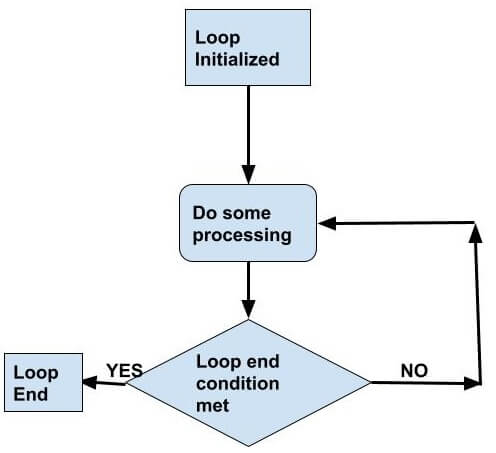Tutorials Hut

• # Scala Loops: For, foreach, while and do while

Most programming languages have loop controls and conditions in code, for example Java, C++ etc, similarly Scala also supports many loops. Let’s try to understand loops. Loop is a repetitive block where any code or statement will be executed multiple times until the end condition of loop is met.

Below diagram shows how loops work:## Four types of Scala Loops

1) for loop – for loop is used to repetitively execute a block of code until the end condition of for loop met.

2) foreach – foreach is similar to for loop because it also rules repetitively but foreach is available in collection classes for example List class where a list elements can be repeatedly processed till the end of list reaches.

3) while loop – as name suggest it is a loop but with while which means while certain condition is true/met the loop will continue.

4) do .. while loop – it is similar to while loop only difference is in while loop it will first check condition and then start execution of statements inside loop, so if condition is no met at start, it may never execute any code in loop and skip it but with do while first it will execute the code and then check if condition is met or not, so at least once the loop will execute for sure.

Now let’s see more details and examples of each type of loop in Scala:

## Scala For Loop

Scala for loops runs and executes code inside it as long as the end condition of the loop are not met.

Syntax:

`var i = 0for(i <- start to end) {//code}`

Example:

`object Loops {def main(args: Array[String]): Unit = {var i = 0while(i < 5) {i = i+1println("in while loop value of i ="+i)}}}`

Output of above while loop program:

`in while loop value of i =1in while loop value of i =2in while loop value of i =3in while loop value of i =4in while loop value of i =5`

## Scala Do While Loop

As we discussed previously, the do while is the same as the while loop, but it ensures that it will run once before checking the end condition, so even if end conditions are always true, it will execute your code once before exiting the loop.

Example of  Scala Do While Loop:

`object Loops {def main(args: Array[String]): Unit = {var i = 0do{   i = i+1   println("in do while loop value of i ="+i)   }while(i < 5)  }}`

Output of Above Scala Do While Loop Program:

`in do while loop value of i =1in do while loop value of i =2in do while loop value of i =3in do while loop value of i =4in do while loop value of i =5`

## Scala forearch and Loop On List

Scala supports foreach and for loop on list, check below example:

Example:

`object Loops {  def main(args: Array[String]): Unit = {  val myList = List(1, 2, 3)  for(i <- myList) { // simple for   println("for loop"+i)} myList.foreach(println) // for each  }}`

Output

`for loop1for loop2for loop3123`

## Next Article

• Scala functions, closure, higher order functions, partial function, currying